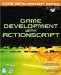# The Tank Demo

 [ LiB ]

Ever wonder how to make your character move in any direction, not just the screen's up, down, left, and right directions? Well, now you can.

The following listing was taken from demo GDA_PROG11.5.fla; it allows you to move a little tank all over the screen. I bet you didn't realize it took some math to move a tank, eh? Check out the following listing.

` // Game Development with ActionScript // By Lewis Moronta (c) 2003 // This program demonstrates // how to use the sine and cosine // trig functions to allow the user // to rotate the main player and // move in which ever direction he // wants. Feel free to modify. // Setup old faithful... onClipEvent(enterFrame) {   // Initialize our velocity variables   var yv = 0;   var xv = 0;      // Check if the user wants to go left     if (Key.isDown(Key.LEFT)) {       // Rotate counter-clockwise         _rotation -= 10;     }     // If we are going rotate, we rotate that way     if (Key.isDown(Key.RIGHT)) {       _rotation += 10;     }     // When the player is ready to move foward     // calculate which way is forward and then     // finally move that way... with the help     // of trigonometry...     if (Key.isDown(Key.UP)) {           // Calculate the angle we are facing...           var angle = this._rotation * (Math.PI/180);           // Calculate the X coordinate for our next           // position in our velocity map           xv += Math.sin(angle)*10;           // Caclulate the Y coordinate for our next position        yv -= Math.cos(angle)*10;     }     // Finally move the player along...     _y += yv;     _x += xv; } `

Just in case you couldn't find the code in the timeline: I embedded it within the tank Movie Clip.

One of the first things I did in this code was set up our old event handler onClipEvent(enterFrame) .

` // Setup old faithful... onClipEvent(enterFrame) { `

As part of the movement mechanism, I have set up two velocity variables that will store the next position to which the tank can jump. You'll see how soon.

` // Initialize our velocity variables   var yv = 0;   var xv = 0; `

The next thing I did was check whether the user pressed the left arrow keyif it was pressed, then the object is rotated counter-clockwise 10 degrees.

` if (Key.isDown(Key.LEFT)) {     // Rotate counter-clockwise         _rotation -= 10; } `

I did something similar with the right key, but this time it rotates clockwise 10 degrees.

` if (Key.isDown(Key.RIGHT)) {     _rotation += 10; } `

It would be kind of hard to detect when the user wants the tank to go forward without the code, so I set up a similar if structure. The only difference is that this one is more complicated.

` if (Key.isDown(Key.UP)) { `

The first thing I did was calculate the angle, in radians, of the position we were in.

` var angle = this._rotation * (Math.PI/180); `

After figuring this out, I went ahead and figured out that the projected point is 10 pixels away. I used sine and cosine for that.

` // Calculate the X coordinate for our next // position in our velocity map xv += Math.sin(angle)*10; // Caclulate the Y coordinate for our next position yv -= Math.cos(angle)*10; `

Notice that the yv value is being subtractedthis is because your monitor's coordinates have y starting at (0, 0) from the upper left. The cosine function graph is on a Cartesian plane, which has y getting higher as you go up. On a monitor, y goes higher when you go down, thus the negative sign.

The next thing I did was very importantif this wasn't in the code, you wouldn't see any locomotion:

` // Finally move the player along...   _y += yv;   _x += xv; `

As new yv and xv values are being calculated on every frame, it's easy to rotate and move the tank to another rotation by calculating new values for them. Cool, right?

 [ LiB ]Game Development with ActionScript
ISBN: 1592001106
EAN: 2147483647
Year: 2004
Pages: 162
Authors: Lewis Moronta